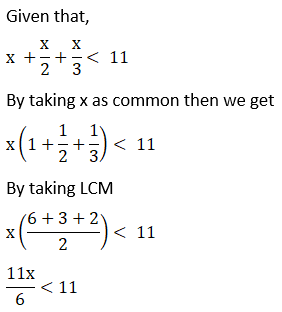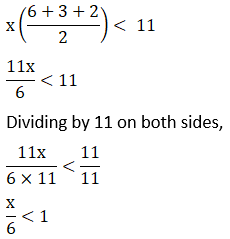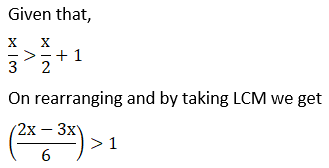• +91 9971497814
• info@interviewmaterial.com

# Chapter 6 Linear Inequalities Ex-6.1 Interview Questions Answers

### Related Subjects

Question 1 :
Solve 24x < 100, when
(i) x is a natural number.
(ii) x is an integer.

(i) Given that 24x < 100
Now we have to divide the inequality by 24 then we get x < 25/6
Now when x is a natural integer then
It is clear that the only natural number less than 25/6 are 1, 2, 3, 4.
Thus, 1, 2, 3, 4 will be the solution of the given inequality when x is a natural number.
Hence {1, 2, 3, 4} is the solution set.
(ii) Given that 24x < 100
Now we have to divide the inequality by 24 then we get x < 25/6
now when x is an integer then
It is clear that the integer number less than 25/6 are…-1, 0, 1, 2, 3, 4.
Thus, solution of 24 x < 100 are…,-1, 0, 1, 2, 3, 4, when x is an integer.
Hence {…, -1, 0, 1, 2, 3, 4} is the solution set.

Question 2 :
Solve – 12x > 30, when
(i) x is a natural number.
(ii) x is an integer.

(i) Given that, – 12x > 30
Now by dividing the inequality by -12 on both sides we get, x < -5/2
When x is a natural integer then
It is clear that there is no natural number less than -2/5 because -5/2 is a negative number and natural numbers are positive numbers.
Therefore there would be no solution of the given inequality when x is a natural number.
(ii) Given that, – 12x > 30
Now by dividing the inequality by -12 on both sides we get, x < -5/2
When x is an integer then
It is clear that the integer number less than -5/2 are…, -5, -4, – 3
Thus, solution of – 12x > 30 is …,-5, -4, -3, when x is an integer.
Therefore the solution set is {…, -5, -4, -3}

Question 3 :
Solve 5x – 3 < 7, when
(i) x is an integer
(ii) x is a real number

(i) Given that, 5x – 3 < 7
Now by adding 3 both side we get,
5x – 3 + 3 < 7 + 3
Above inequality becomes
5x < 10
Again by dividing both sides by 5 we get,
5x/5 < 10/5
x < 2
When x is an integer then
It is clear that that the integer number less than 2 are…, -2, -1, 0, 1.
Thus, solution of 5x – 3 < 7 is …,-2, -1, 0, 1, when x is an integer.
Therefore the solution set is {…, -2, -1, 0, 1}
(ii) Given that, 5x – 3 < 7
Now by adding 3 both side we get,
5x – 3 + 3 < 7 + 3
Above inequality becomes
5x < 10
Again by dividing both sides by 5 we get,
5x/5 < 10/5
x < 2
When x is a real number then
It is clear that the solutions of 5x – 3 < 7 will be given by x < 2 which states that all the real numbers that are less than 2.
Hence the solution set is x ∈ (-∞, 2)

Question 4 :
Solve 3x + 8 >2, when
(i) x is an integer.
(ii) x is a real number.

(i) Given that, 3x + 8 > 2
Now by subtracting 8 from both sides we get,
3x + 8 – 8 > 2 – 8
The above inequality becomes,
3x > – 6
Again by dividing both sides by 3 we get,
3x/3 > -6/3
Hence x > -2
When x is an integer then
It is clear that the integer number greater than -2 are -1, 0, 1, 2,…
Thus, solution of 3x + 8 > 2is -1, 0, 1, 2,… when x is an integer.
Hence the solution set is {-1, 0, 1, 2,…}
(ii) Given that, 3x + 8 > 2
Now by subtracting 8 from both sides we get,
3x + 8 – 8 > 2 – 8
The above inequality becomes,
3x > – 6
Again by dividing both sides by 3 we get,
3x/3 > -6/3
Hence x > -2
When x is a real number.
It is clear that the solutions of 3x + 8 >2 will be given by x > -2 which states that all the real numbers that are greater than -2.
Therefore the solution set is x ∈ (-2, ∞)
Solve the inequalities in Exercises 5 to 16 for real x.

Question 5 : 4x + 3 < 5x + 7

Given that, 4x + 3 < 5x + 7
Now by subtracting 7 from both the sides, we get
4x + 3 – 7 < 5x + 7 – 7
The above inequality becomes,
4x – 4 < 5x
Again by subtracting 4x from both the sides,
4x – 4 – 4x < 5x – 4x
x > – 4
∴The solutions of the given inequality are defined by all the real numbers greater than -4.
Required solution set is (-4, ∞)

Question 6 : 3x – 7 > 5x – 1

Given that,
3x – 7 > 5x – 1
Now by adding 7 to both the sides, we get
3x – 7 +7 > 5x – 1 + 7
3x > 5x + 6
Again by subtracting 5x from both the sides,
3x – 5x > 5x + 6 – 5x
-2x > 6
Dividing both sides by -2 to simplify we get
-2x/-2 < 6/-2
x < -3
∴ The solutions of the given inequality are defined by all the real numbers less than -3.
Hence the required solution set is (-∞, -3)

Question 7 : 3(x – 1) ≤ 2 (x – 3)

Given that, 3(x – 1) ≤ 2 (x – 3)
By multiplying above inequality can be written as
3x – 3 ≤ 2x – 6
Now by adding 3 to both the sides, we get
3x – 3+ 3 ≤ 2x – 6+ 3
3x ≤ 2x – 3
Again by subtracting 2x from both the sides,
3x – 2x ≤ 2x – 3 – 2x
x ≤ -3
Therefore the solutions of the given inequality are defined by all the real numbers less than or equal to -3.
Hence the required solution set is (-∞, -3]

Question 8 : 3 (2 – x) ≥ 2 (1 – x)

Given that, 3 (2 – x) ≥ 2 (1 – x)
By multiplying we get
6 – 3x ≥ 2 – 2x
Now by adding 2x to both the sides,
6 – 3x + 2x ≥ 2 – 2x + 2x
6 – x ≥ 2
Again by subtracting 6 from both the sides, we get
6 – x – 6 ≥ 2 – 6
– x ≥ – 4
Multiplying throughout inequality by negative sign we get
x ≤ 4
∴ The solutions of the given inequality are defined by all the real numbers greater than or equal to 4.
Hence the required solution set is (- ∞, 4]

Question 9 : x + x/2 + x/3 < 11x < 6
The solutions of the given inequality are defined by all the real numbers less than 6.
Hence the solution set is (-∞, 6)

Question 10 : x/3 > x/2 + 1– x/6 > 1
– x > 6
x < – 6
∴ The solutions of the given inequality are defined by all the real numbers less than – 6.
Hence the required solution set is (-∞, -6)

Todays Deals### Chapter 6 Linear Inequalities Ex-6.1 Contributorskrishan

Name:
Email:

# Latest News# 9000 interview questions in different categories